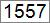Hello Learner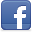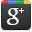Contributor
Stats
 Views : 19593 Comments : 0 Rating : 0
Actions

# .NET program to find greatest of three numbers

By Ashok Nalam on 28 Jun 2013 | Category: C# | Tagged: number .NET
The program finds the greatest of three numbers and then prints the number which is the greatest. If all 3 input numbers are same then it prints "Entered Numbers are not Distinct.".Add Comment   |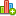Rate this resource

The program finds greatest of three numbers and then prints the number which is the greatest. If all 3 input numbers are same then it prints "Entered Numbers are not Distinct.".

#### Program:

```namespace DotNetMirror
{
class GreatestOfThreeNumbers
{
static void Main()
{
int number1, number2, number3;
Console.Write("Enter three numbers (followed by Enter key): ");
if (number1 > number2 && number1 > number3) // compare number 1 with 2 & 3
{
Console.Write("Number {0} is largest.", number1);
}
else if (number2 > number1 && number2 > number3) // compare number 2 with 1 & 3
{
Console.Write("Number {0} is largest.", number2);
}
else if (number3 > number1 && number3 > number2) // compare number 3 with 1 & 2
{
Console.Write("Number {0} is largest.", number3);
}
else //all 3 numbers are same
{
Console.Write("Entered Numbers are not Distinct.");
}
}
}
}```

#### Output: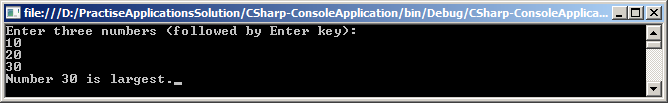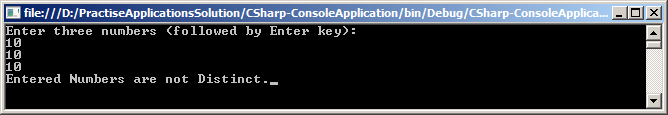Note: If you want find greatest number for more than 3 numbers, then conditions will be more and logic becomes complex. In such scenario you can go for array comparison.Add CommentRate this resource
 Name Email WebSite